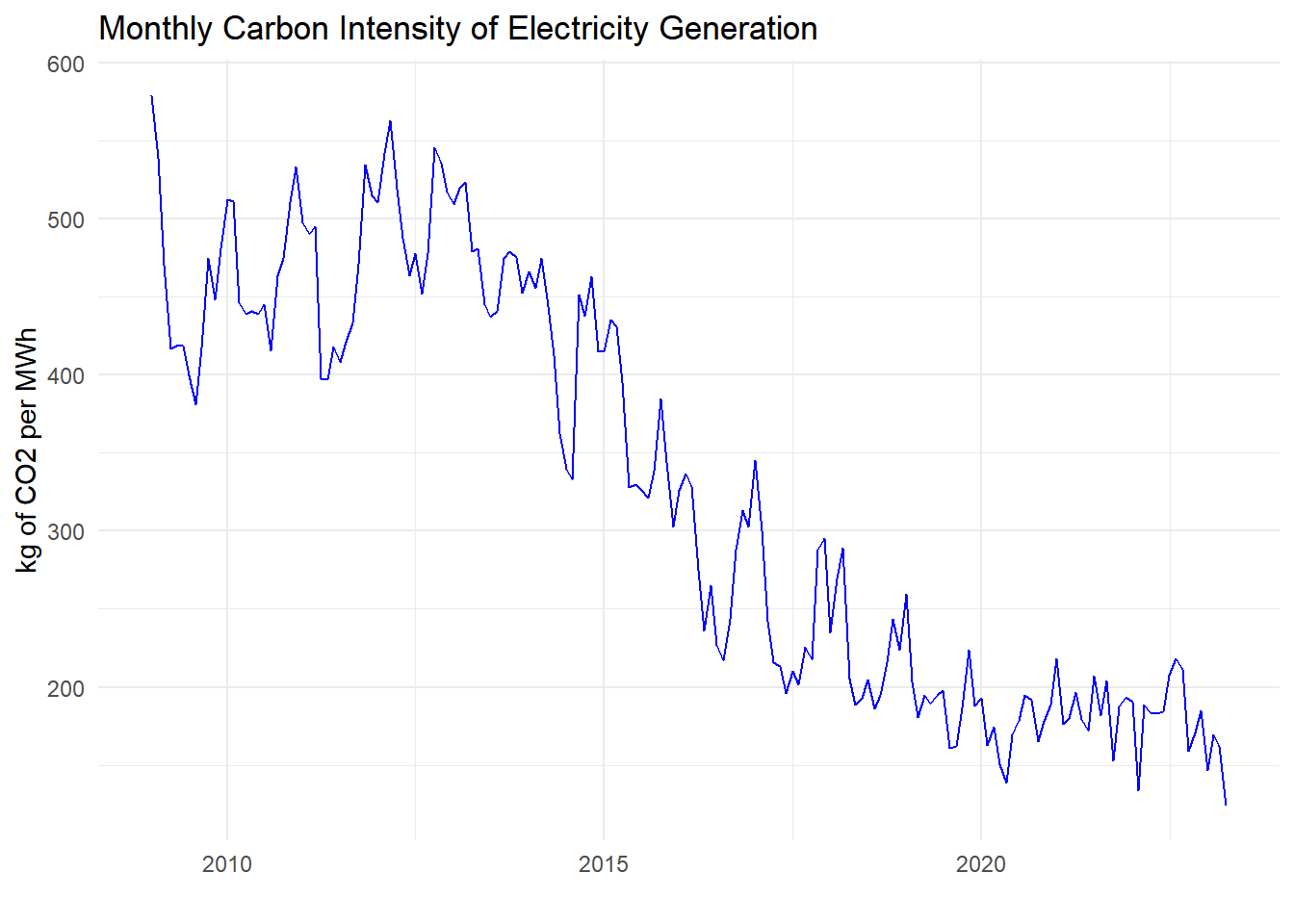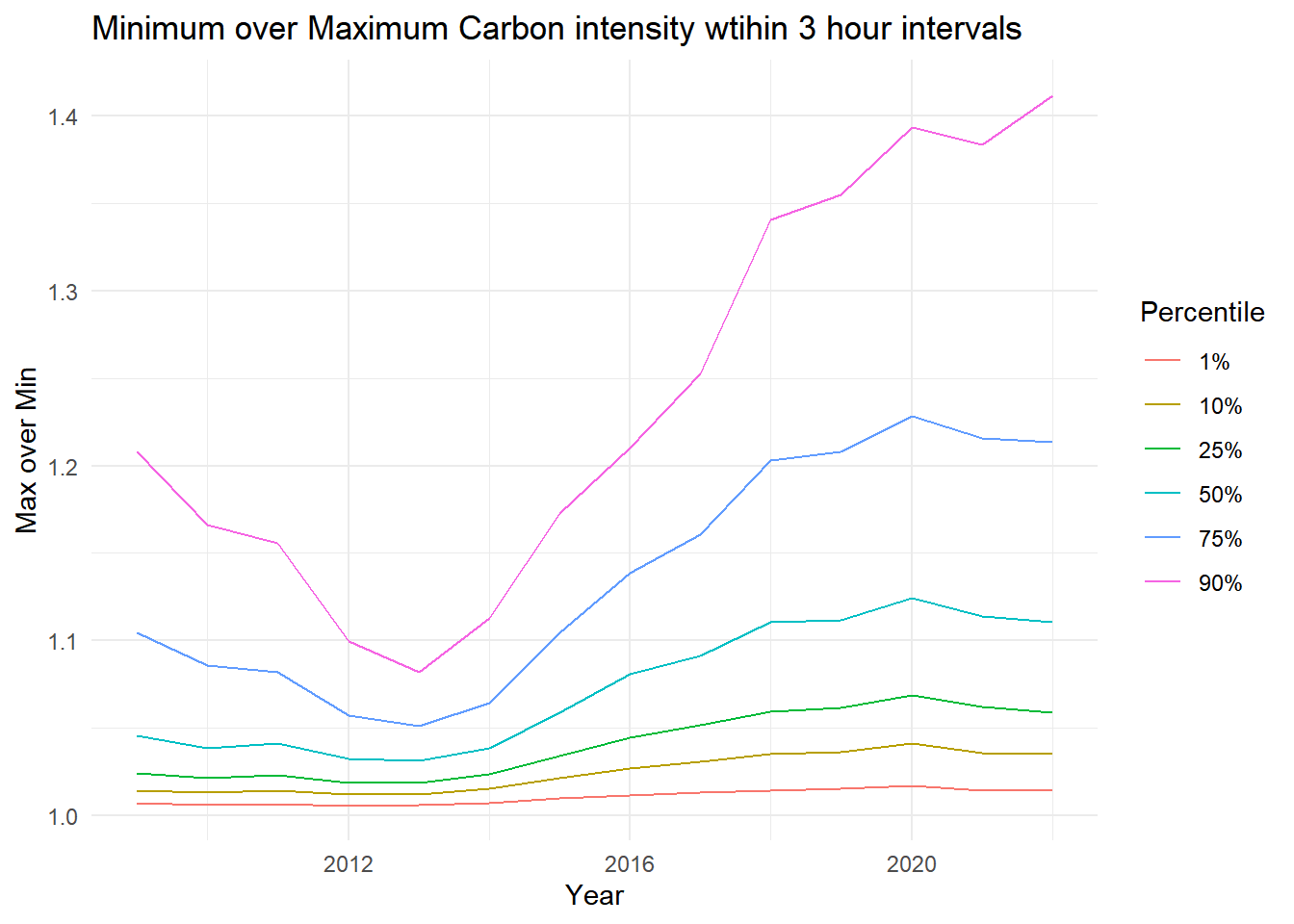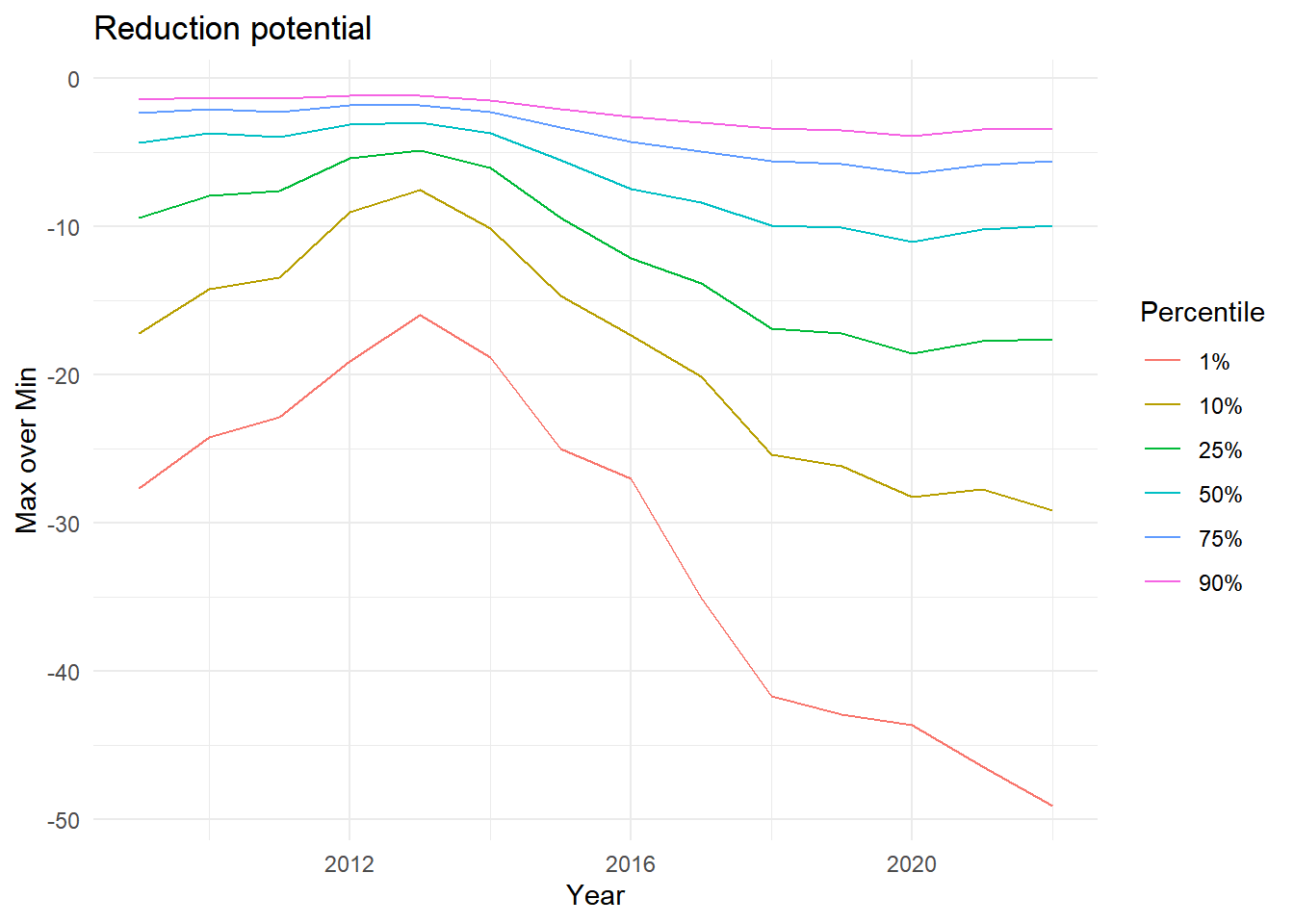# Carbon intensity of electricity in the UK

The carbon intensity of UK electricity generation has dramatically reduced in recent years primarily due to an ever larger share of renewable energy generation.A side effect of this is that carbon intensity is more variable within short periods of time. For instance the figure below shows how much carbon intensity differs within moving 3 hour time intervals by plotting various quantiles of the ratio between minimum and maximum carbon intensity:For instance, in 2009 (at the beginning of our sample period) the ratio between maximum and minimum carbon intensity was less than 1.1 in 75% of all 3 hour periods. By 2020 this 75% quatnile has risen to 1.2 implying in turn that there was a more than 20% difference in 25% of 3 hour periods. In 10% of 3 hour periods there was indeed a more than 40% difference.

This potentially implies that even small changes in the time of consuming electricity could make a big difference in terms of the amount of carbon associated with that consumption. To illustrate this the figure below reports the percentage difference of carbon pollution implied by consuming at the least vs the most carbon intensive time point within a three hour period. Again we look at the distribution across all 3 hour periods within a year.Hence, the Figure shows for instance that in 2009, in 25% of 3 hour periods consuming a kWh of electricity at the least polluting time point in that 3 hour period would be at least 10% less polluting than consuming at the most polluting time point. In 2022 it would be 20% less polluting. In 10% of case it be 30% (or more) less polluting.

Hence, there is potentially a lot more potential for load balancing. However, before we can say that for sure we need to do more analysis, becasue the average carbon intensity at every time point does not necessarily reflect the causal impact of moving a given amount of consumption from one time period to the next. To assess that we need to work out the marginal carbon intensities.

POWBAL researchers are currently working on a new methodology to do this. Stay tuned for new results soon.